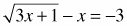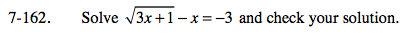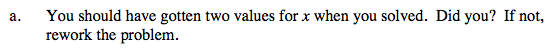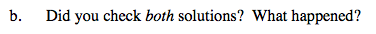Home > A2C > Chapter 7 > Lesson 7.3.1 > Problem7-162

7-162.
1. Solveand check your solution. Homework Help ✎

1. You should have gotten two values for x when you solved. Did you? If not, rework the problem.

2. Did you check both solutions? What happened?Add x to both sides. Then square both sides.
You will need to use the Distributive Property.

Set the equation equal to 0. You will need to factor or use the Quadratic Formula to solve.

x = 1 or x = 8One of the two solutions is extraneous (does not check).